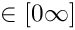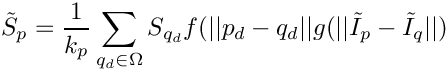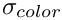Point Cloud Library (PCL)  1.13.1-dev
pcl::BilateralUpsampling< PointInT, PointOutT > Class Template Reference

Bilateral filtering implementation, based on the following paper: More...

#include <pcl/surface/bilateral_upsampling.h>Inheritance diagram for pcl::BilateralUpsampling< PointInT, PointOutT >:Collaboration diagram for pcl::BilateralUpsampling< PointInT, PointOutT >:

## Public Types

using Ptr = shared_ptr< BilateralUpsampling< PointInT, PointOutT > >

using ConstPtr = shared_ptr< const BilateralUpsampling< PointInT, PointOutT > >

using PointCloudOut = pcl::PointCloud< PointOutT >Public Types inherited from pcl::CloudSurfaceProcessing< PointInT, PointOutT >
using Ptr = shared_ptr< CloudSurfaceProcessing< PointInT, PointOutT > >

using ConstPtr = shared_ptr< const CloudSurfaceProcessing< PointInT, PointOutT > >Public Types inherited from pcl::PCLBase< PointInT >
using PointCloud = pcl::PointCloud< PointInT >

using PointCloudPtr = typename PointCloud::Ptr

using PointCloudConstPtr = typename PointCloud::ConstPtr

using PointIndicesPtr = PointIndices::Ptr

using PointIndicesConstPtr = PointIndices::ConstPtr

## Public Member Functions

BilateralUpsampling ()
Constructor. More...

void setWindowSize (int window_size)
Method that sets the window size for the filter. More...

int getWindowSize () const
Returns the filter window size. More...

void setSigmaColor (const float &sigma_color)
Method that sets the sigma color parameter. More...

float getSigmaColor () const
Returns the current sigma color value. More...

Method that sets the sigma depth parameter. More...

Returns the current sigma depth value. More...

void setProjectionMatrix (const Eigen::Matrix3f &projection_matrix)
Method that sets the projection matrix to be used when unprojecting the points in the depth image back to (x,y,z) positions. More...

Eigen::Matrix3f getProjectionMatrix () const
Returns the current projection matrix. More...

void process (pcl::PointCloud< PointOutT > &output) override
Method that does the actual processing on the input cloud. More...Public Member Functions inherited from pcl::CloudSurfaceProcessing< PointInT, PointOutT >
CloudSurfaceProcessing ()
Constructor. More...

~CloudSurfaceProcessing () override=default
Empty destructor. More...Public Member Functions inherited from pcl::PCLBase< PointInT >
PCLBase ()
Empty constructor. More...

PCLBase (const PCLBase &base)
Copy constructor. More...

virtual ~PCLBase ()=default
Destructor. More...

virtual void setInputCloud (const PointCloudConstPtr &cloud)
Provide a pointer to the input dataset. More...

PointCloudConstPtr const getInputCloud () const
Get a pointer to the input point cloud dataset. More...

virtual void setIndices (const IndicesPtr &indices)
Provide a pointer to the vector of indices that represents the input data. More...

virtual void setIndices (const IndicesConstPtr &indices)
Provide a pointer to the vector of indices that represents the input data. More...

virtual void setIndices (const PointIndicesConstPtr &indices)
Provide a pointer to the vector of indices that represents the input data. More...

virtual void setIndices (std::size_t row_start, std::size_t col_start, std::size_t nb_rows, std::size_t nb_cols)
Set the indices for the points laying within an interest region of the point cloud. More...

IndicesPtr getIndices ()
Get a pointer to the vector of indices used. More...

IndicesConstPtr const getIndices () const
Get a pointer to the vector of indices used. More...

const PointInT & operator[] (std::size_t pos) const
Override PointCloud operator[] to shorten code. More...

## Public Attributes

Eigen::Matrix3f KinectVGAProjectionMatrix

Eigen::Matrix3f KinectSXGAProjectionMatrix

## Protected Member Functions

void performProcessing (pcl::PointCloud< PointOutT > &output) override
Abstract cloud processing method. More...

void computeDistances (Eigen::MatrixXf &val_exp_depth, Eigen::VectorXf &val_exp_rgb)
Computes the distance for depth and RGB. More...Protected Member Functions inherited from pcl::PCLBase< PointInT >
bool initCompute ()
This method should get called before starting the actual computation. More...

bool deinitCompute ()
This method should get called after finishing the actual computation. More...Protected Attributes inherited from pcl::PCLBase< PointInT >
PointCloudConstPtr input_
The input point cloud dataset. More...

IndicesPtr indices_
A pointer to the vector of point indices to use. More...

bool use_indices_
Set to true if point indices are used. More...

bool fake_indices_
If no set of indices are given, we construct a set of fake indices that mimic the input PointCloud. More...

## Detailed Description

### template<typename PointInT, typename PointOutT> class pcl::BilateralUpsampling< PointInT, PointOutT >

Bilateral filtering implementation, based on the following paper:

• Kopf, Johannes and Cohen, Michael F. and Lischinski, Dani and Uyttendaele, Matt - Joint Bilateral Upsampling,
• ACM Transactions in Graphics, July 2007

Takes in a colored organized point cloud (i.e. PointXYZRGB or PointXYZRGBA), that might contain nan values for the depth information, and it will return an upsampled version of this cloud, based on the formula:where S is the depth image, I is the RGB image and f and g are Gaussian functions centered at 0 and with standard deviationsandDefinition at line 63 of file bilateral_upsampling.h.

## ◆ ConstPtr

template<typename PointInT , typename PointOutT >
 using pcl::BilateralUpsampling< PointInT, PointOutT >::ConstPtr = shared_ptr >

Definition at line 67 of file bilateral_upsampling.h.

## ◆ PointCloudOut

template<typename PointInT , typename PointOutT >
 using pcl::BilateralUpsampling< PointInT, PointOutT >::PointCloudOut = pcl::PointCloud

Definition at line 75 of file bilateral_upsampling.h.

## ◆ Ptr

template<typename PointInT , typename PointOutT >
 using pcl::BilateralUpsampling< PointInT, PointOutT >::Ptr = shared_ptr >

Definition at line 66 of file bilateral_upsampling.h.

## ◆ BilateralUpsampling()

template<typename PointInT , typename PointOutT >
 pcl::BilateralUpsampling< PointInT, PointOutT >::BilateralUpsampling ( )
inline

Constructor.

Definition at line 80 of file bilateral_upsampling.h.

## ◆ computeDistances()

template<typename PointInT , typename PointOutT >
 void pcl::BilateralUpsampling< PointInT, PointOutT >::computeDistances ( Eigen::MatrixXf & val_exp_depth, Eigen::VectorXf & val_exp_rgb )
protected

Computes the distance for depth and RGB.

Parameters
 [out] val_exp_depth distance values for depth [out] val_exp_rgb distance values for RGB

Definition at line 156 of file bilateral_upsampling.hpp.

## ◆ getProjectionMatrix()

template<typename PointInT , typename PointOutT >
 Eigen::Matrix3f pcl::BilateralUpsampling< PointInT, PointOutT >::getProjectionMatrix ( ) const
inline

Returns the current projection matrix.

Definition at line 133 of file bilateral_upsampling.h.

## ◆ getSigmaColor()

template<typename PointInT , typename PointOutT >
 float pcl::BilateralUpsampling< PointInT, PointOutT >::getSigmaColor ( ) const
inline

Returns the current sigma color value.

Definition at line 111 of file bilateral_upsampling.h.

template<typename PointInT , typename PointOutT >
 float pcl::BilateralUpsampling< PointInT, PointOutT >::getSigmaDepth ( ) const
inline

Returns the current sigma depth value.

Definition at line 121 of file bilateral_upsampling.h.

## ◆ getWindowSize()

template<typename PointInT , typename PointOutT >
 int pcl::BilateralUpsampling< PointInT, PointOutT >::getWindowSize ( ) const
inline

Returns the filter window size.

Definition at line 101 of file bilateral_upsampling.h.

## ◆ performProcessing()

template<typename PointInT , typename PointOutT >
 void pcl::BilateralUpsampling< PointInT, PointOutT >::performProcessing ( pcl::PointCloud< PointOutT > & output )
overrideprotectedvirtual

Abstract cloud processing method.

Definition at line 88 of file bilateral_upsampling.hpp.

## ◆ process()

template<typename PointInT , typename PointOutT >
 void pcl::BilateralUpsampling< PointInT, PointOutT >::process ( pcl::PointCloud< PointOutT > & output )
overridevirtual

Method that does the actual processing on the input cloud.

Parameters
 [out] output the container of the resulting upsampled cloud

Reimplemented from pcl::CloudSurfaceProcessing< PointInT, PointOutT >.

Definition at line 50 of file bilateral_upsampling.hpp.

## ◆ setProjectionMatrix()

template<typename PointInT , typename PointOutT >
 void pcl::BilateralUpsampling< PointInT, PointOutT >::setProjectionMatrix ( const Eigen::Matrix3f & projection_matrix )
inline

Method that sets the projection matrix to be used when unprojecting the points in the depth image back to (x,y,z) positions.

Note
There are 2 matrices already set in the class, used for the 2 modes available for the Kinect. They are tuned to be the same as the ones in the OpenNiGrabber
Parameters
 [in] projection_matrix the new projection matrix to be set

Definition at line 129 of file bilateral_upsampling.h.

## ◆ setSigmaColor()

template<typename PointInT , typename PointOutT >
 void pcl::BilateralUpsampling< PointInT, PointOutT >::setSigmaColor ( const float & sigma_color )
inline

Method that sets the sigma color parameter.

Parameters
 [in] sigma_color the new value to be set

Definition at line 107 of file bilateral_upsampling.h.

template<typename PointInT , typename PointOutT >
 void pcl::BilateralUpsampling< PointInT, PointOutT >::setSigmaDepth ( const float & sigma_depth )
inline

Method that sets the sigma depth parameter.

Parameters
 [in] sigma_depth the new value to be set

Definition at line 117 of file bilateral_upsampling.h.

## ◆ setWindowSize()

template<typename PointInT , typename PointOutT >
 void pcl::BilateralUpsampling< PointInT, PointOutT >::setWindowSize ( int window_size )
inline

Method that sets the window size for the filter.

Parameters
 [in] window_size the given window size

Definition at line 97 of file bilateral_upsampling.h.

## ◆ KinectSXGAProjectionMatrix

template<typename PointInT , typename PointOutT >
 Eigen::Matrix3f pcl::BilateralUpsampling< PointInT, PointOutT >::KinectSXGAProjectionMatrix

Definition at line 77 of file bilateral_upsampling.h.

## ◆ KinectVGAProjectionMatrix

template<typename PointInT , typename PointOutT >
 Eigen::Matrix3f pcl::BilateralUpsampling< PointInT, PointOutT >::KinectVGAProjectionMatrix

Definition at line 77 of file bilateral_upsampling.h.

The documentation for this class was generated from the following files: